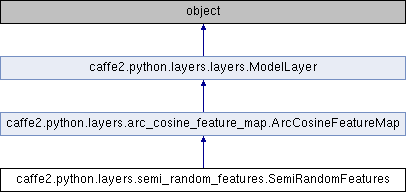Caffe2 - Python API A deep learning, cross platform ML framework
caffe2.python.layers.semi_random_features.SemiRandomFeatures Class Reference
Inheritance diagram for caffe2.python.layers.semi_random_features.SemiRandomFeatures:## Public Member Functions

def __init__ (self, model, input_record, output_dims, s=1, scale_random=1.0, scale_learned=1.0, weight_init_random=None, bias_init_random=None, weight_init_learned=None, bias_init_learned=None, weight_optim=None, bias_optim=None, set_weight_as_global_constant=False, name='semi_random_features', kwargs)Public Member Functions inherited from caffe2.python.layers.arc_cosine_feature_map.ArcCosineFeatureMap
def __init__ (self, model, input_record, output_dims, s=1, scale=1.0, weight_init=None, bias_init=None, weight_optim=None, bias_optim=None, set_weight_as_global_constant=False, initialize_output_schema=True, name='arc_cosine_feature_map', kwargs)Public Member Functions inherited from caffe2.python.layers.layers.ModelLayer
def __init__ (self, model, prefix, input_record, predict_input_record_fields=None, tags=None, kwargs)

def get_type (self)

def predict_input_record (self)

def input_record (self)

def predict_output_schema (self)

def predict_output_schema (self, output_schema)

def output_schema (self)

def output_schema (self, output_schema)

def get_parameters (self)

def get_fp16_compatible_parameters (self)

def get_memory_usage (self)

def create_param (self, param_name, shape, initializer, optimizer, ps_param=None, regularizer=None)

def get_next_blob_reference (self, name)

def add_operators (self, net, init_net=None, context=InstantiationContext.TRAINING)

def export_output_for_metrics (self)

def export_params_for_metrics (self)

## Public Attributes

input_record_full

input_record_random

output_schema

stddevPublic Attributes inherited from caffe2.python.layers.arc_cosine_feature_map.ArcCosineFeatureMap
params

model

set_weight_as_global_constant

input_dims

output_schema

output_dims

s

stddev

random_w

random_bPublic Attributes inherited from caffe2.python.layers.layers.ModelLayer
name

model

kwargs

request_only

precomputation_request_only

precomputation_object_only

eval_output_schema

tags

params

## Detailed Description

```Implementation of the semi-random kernel feature map.

Applies H(x_rand) * x_rand^s * x_learned, where
H is the Heaviside step function,
x_rand is the input after applying FC with randomized parameters,
and x_learned is the input after applying FC with learnable parameters.

If using multilayer model with semi-random layers, then input and output records
should have a 'full' and 'random' Scalar. The random Scalar will be passed as
input to process the random features.

https://arxiv.org/pdf/1702.08882.pdf

Inputs :
output_dims -- dimensions of the output vector
s -- if s == 0, will obtain linear semi-random features;
else if s == 1, will obtain squared semi-random features;
else s >= 2, will obtain higher order semi-random features
scale_random -- amount to scale the standard deviation
(for random parameter initialization when weight_init or
bias_init hasn't been specified)
scale_learned -- amount to scale the standard deviation
(for learned parameter initialization when weight_init or
bias_init hasn't been specified)

weight_init_random -- initialization distribution for random weight parameter
(if None, will use Gaussian distribution)
bias_init_random -- initialization distribution for random bias pararmeter
(if None, will use Uniform distribution)
weight_init_learned -- initialization distribution for learned weight parameter
(if None, will use Gaussian distribution)
bias_init_learned -- initialization distribution for learned bias pararmeter
(if None, will use Uniform distribution)
weight_optim -- optimizer for weight params for learned features
bias_optim -- optimizer for bias param for learned features

set_weight_as_global_constant -- if True, initialized random parameters
will be constant across all distributed
instances of the layer
```

Definition at line 11 of file semi_random_features.py.

The documentation for this class was generated from the following file: